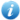English  |  正體中文  |  简体中文  |  Items with full text/Total items : 70585/70585 (100%) Visitors : 23256417      Online Users : 513
 Scope All of NCUIR 理學院    數學研究所       --期刊論文Tips: please add "double quotation mark" for query phrases to get precise resultsplease goto advance search for comprehansive author search Adv. Search
 Home ‧ Login ‧ Upload ‧ Help ‧ About ‧ AdministerNCU Institutional Repository > 理學院 > 數學研究所 > 期刊論文 >  Item 987654321/27952

 Please use this identifier to cite or link to this item: `http://ir.lib.ncu.edu.tw/handle/987654321/27952`

 Title: Mutually independent hamiltonian cycles for the pancake graphs and the star graphs Authors: Lin,CK;Tan,JJM;Huang,HM;Hsu,DF;Hsu,LH Contributors: 數學研究所 Keywords: VERTEX-SYMMETRIC GRAPHS;CUBIC CAYLEY-GRAPHS;INTERCONNECTION NETWORKS;FAULT-DIAMETER;PATHS;LACEABILITY;TREES Date: 2009 Issue Date: 2010-06-29 19:38:45 (UTC+8) Publisher: 中央大學 Abstract: A hamiltonian cycle C of a graph G is an ordered set < u(1), u(2,) ..., u(n(G)), u(1)> of vertices such that u(i) not equal u(j) for i not equal j and u(i) is adjacent to u(i+1) for every i is an element of {1, 2, ..., n(G) - 1} and u(n(G)) is adjacent to u(1), where n(G) is the order of G. The vertex u(1) is the starting vertex and u(i) is the ith vertex of C. Two hamiltonian cycles C-1 = < u(1), u(2), ..., u(n(G)), u(1)> and C-2 = < v(1), v(2), ..., v(n(G)), v(1)> of G are independent if u(1) = v(1) and u(i) not equal v(i) for every i is an element of {2, 3, ..., n(G)}. A set of hamiltonian cycles {C-1, C-2, ..., C-k} of G is mutually independent if its elements are pairwise independent. The mutually independent hamiltonicity IHC(G) of a graph G is the maximum integer k such that for any vertex u of G there exist k mutually independent hamiltonian cycles of G starting at u. In this paper, the mutually independent hamiltonicity is considered for two families of Cayley graphs, the n-dimensional pancake graphs P-n and the n-dimensional star graphs S-n. It is proven that IHC(P-3) = 1, IHC(P-n) = n - 1 if n >= 4, IHC(S-n) = n - 2 if n is an element of {3, 4} and IHC(S-n) = n - 1 if n >= 5. (C) 2009 Elsevier B.V. All rights reserved. Relation: DISCRETE MATHEMATICS Appears in Collections: [數學研究所] 期刊論文

Files in This Item:

File Description SizeFormat
index.html0KbHTML714View/Open